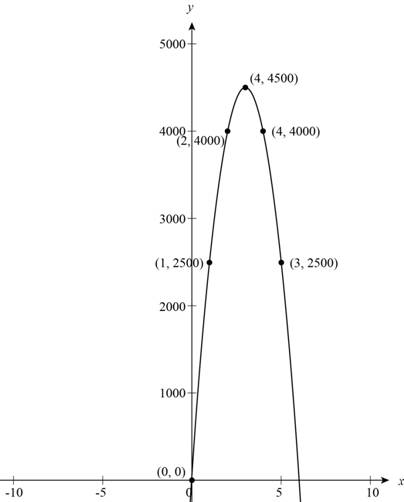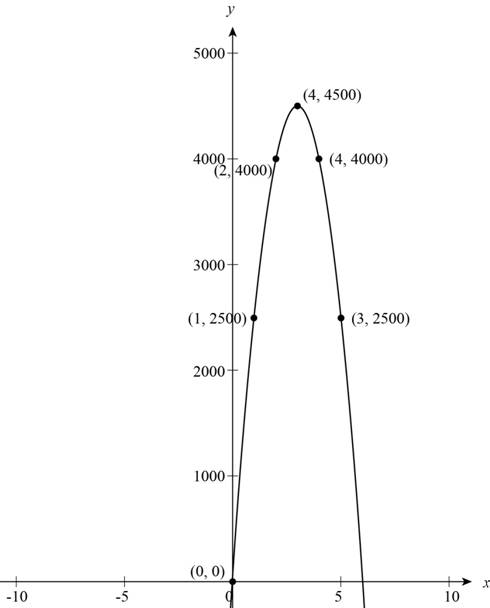# The value of R ( 2 ) and R ( 4 ) and describe represented values.### Precalculus: Mathematics for Calcu...

6th Edition
Stewart + 5 others
Publisher: Cengage Learning
ISBN: 9780840068071### Precalculus: Mathematics for Calcu...

6th Edition
Stewart + 5 others
Publisher: Cengage Learning
ISBN: 9780840068071

#### Solutions

Chapter 2, Problem 4T

(a)

To determine

## To find: The value of R(2 ) and R(4) and describe represented values.

Expert Solution

The value of R(x) at R(2) is 4000 and at R(4) is 2000  Value represent the total sale revenue with prices of $2 and$4 .

### Explanation of Solution

Given:

The given function is,

R(x)=500x2+3000x

Calculations:

The given function is,

R(x)=500x2+3000x

Substitute 2 for x in the above function to find the value of R(2) ,

R(2)=500×(2)2+3000×2=500×4+3000×2=4000

The value of R(x) at R(2) is 4000 .

Substitute 4 for x in the above function to find the value of R(4) ,

R(4)=500×(4)2+3000×4=500×16+3000×2=2000

The value of R(x) at R(4) is 2000 .

Thus, the value of R(x) at R(2) is 4000 and at R(4) is 2000 . Value represent the total sale revenue with prices of $2 and$4 .

(b)

To determine

Expert Solution

### Explanation of Solution

The given function is,

R(x)=500x2+3000x

Substitute some value of x and make a table for x and R(x).

 x R(x) 0 0 1 2500 2 4000 3 4500 4 4000 5 2500

The graph of function R(x) is shown in Figure (1),Figure (1)

Hence, the revenue increase up to $3 and decreases after$3 .

(c)

To determine

### To find: The maximum revenue, and price.

Expert Solution

The maximum revenue is $4500 and it can achieve at price$3 .

### Explanation of Solution

The given function is,

R(x)=500x2+3000x

The graph of function R(x) is shown in Figure (1).Figure (1)

Form Figure (1), the $4500 is the peak value of the graph at R(3) . Hence, the maximum revenue is$4500 and it can achieve at price \$3 .

### Have a homework question?

Subscribe to bartleby learn! Ask subject matter experts 30 homework questions each month. Plus, you’ll have access to millions of step-by-step textbook answers!